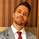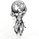צפיות ‎10349‎
10349
// Constructs the trailing ATR stop above or below the price, and switches
// directions when the source price breaks the ATR stop. Uses the Average
// Directional Index ( ADX ) to switch between ATR multipliers. The higher
// multiplier is used when the ADX is rising, and the lower ATR multiplier
// is used with the ADX is falling. This ADX criteria further widens the gap
// between the source price and the trailing ATR stop when the price is trending,
// and lessens the gap between the ATR and the price when then price is not
// trending.
//
// The ATR-ADX stop is effectively a double adapative stop that trails the price,
// by both adapting to the true range of the price, and the average directional
// change. When the stop is below the price (long trade) the value never decreases
// until the price intersects the stop, and it reverses to being above the price
// (short trade). When the stop is above the price it will never increase until
// it is intersected by the price. As the true range and ADX change, the stop
// will move more quickly or more slowly.
//
// Version 2 adds four additional utilities.
//
// First, if the 'Above Threshold' box is checked,the falling (smaller) multiplier
// will be used regardless once the ADX rises above a certain threshold (default > 30).
// The ATR will effectively rise faster once the price enters 'very trendy' mode.
// Typically, ADX > 20/25 is used in classic ADX trading (which I have found
// unprofitable through backtesting). The idea behind this extra multiplier criteria
// is that once the price starts trending 'very' well, a top/bottom is likely near,
// and when that top comes the price will quickly rebound. Experienced traders know
// exactly what I am describing. Play around with an ADX/DI indicator using this
// stop system in tandem and it will be found that many successful trades are entered
// when the market is not trending (i.e. < ADX < 25), and exited once/if the price
// enters 'very trendy' mode above 30 and the ATR changes (stopped out).
//
// Second, heiken-ashi bars can be introduced to the ATR stop system. This is the same
// thing as just switching the chart to Heiken Ashi mode, but without having to change
// the plotting type. I find this useful, so that things like pivot lines can be
// preserved to their correct calculations, while the benefit of heiken ashi bars can
// still be enjoyed. Be advised that with each tinkering that extends the life of the
// ATR trend, the odds of that same trend changing near the top/bottom are reduced,
// so as always there is a trade-off to maximize the time spend in a trade and nailing
// a top or bottom.
//
// Third, Different source prices can be used. I have found that HLC3 is best because
// it keeps the price from being stopped out in very key areas, set as the default.
//
// Fourth, alert conditions are introduced so that the trader can be warned when the
// ATR-ADX changes. These can be used by right clicking the strategy and clicking
// "Add Alert...". Reference the bottom of the script for the names of the alert
// conditions.
//

To show the difference between checking the "Use falling ATR Multiplier if ADX > Threshold" box I have plotted two instances of the Adaptive ATR Indicator using the same settings, except one checks this box and one doesn't. Obviously no two tickers and no two time frames are the same, so it will require some tinkering to get it right. 3.5 Rising and 1.75 Falling seem to be good starting points based on the article link and my own tests.

Cheers.
הערות שחרור: Added multi-timeframe aspect to the script. Input your desired interval (15, 60, 240, D, W, M, 3M, 12M, etc) to view the indicator on an interval that is independent of the chart.

No repainting.
הערות שחרור: Added back in trend change alert conditions.
הערות שחרור: Updated to version 3.

Forced a positive (green) band to be the ceiling of the nearest pip/tick. Forced a negative (red) band to be the floor of the nearest pip/tick. This helps with rounding and intersections.

Added a pip/tick band around the stop for more resistance/support threshold visualizations.
הערות שחרור: Removed unused portions of script.
הערות שחרור: Fixed security function issue.
Another day another dollar.

Telegram: https://t.me/mortdiggiddy
Daily Edge Trading Group: https://www.daily-edge.com
Paypal: https://www.paypal.me/mortdiggiddy
Bitcoin: 3G7icnFJasC7WWShZy5qgczaf2GmkRFkNvinput_len = input(10, title="Length")
input_sym1 = input(title="Symbol1", type=symbol, defval="NSE:HDFCBANK")
//input_sym1_current = input(false, title="Use Current Symbol for Symbol1")
input_sym2 = input(title="Symbol2", type=symbol, defval="NSE:ICICIBANK")
input_sym3 = input(title="Symbol3", type=symbol, defval="NSE:KOTAKBANK")
input_sym4 = input(title="Symbol4", type=symbol, defval="NSE:AXISBANK")
input_sym5 = input(title="Symbol5", type=symbol, defval="NSE:SBIN")
input_sym6 = input(title="Symbol6", type=symbol, defval="NSE:INDUSINDBK")
input_sym7 = input(title="Symbol7", type=symbol, defval="NSE:FEDERALBNK")
input_sym8 = input(title="Symbol8", type=symbol, defval="NSE:YESBANK")
input_sym9 = input(title="Symbol9", type=symbol, defval="NSE:RBLBANK")
input_sym10 = input(title="Symbol10", type=symbol, defval="NSE:BANKBARODA")
no_bank = input(10,title="No of input ticks")

upD = change(high)
downD2 = -change(low)
trurD2 = rma(tr, len)
plusD = fixnan(100 * rma(upD2 > downD2 and upD2 > 0 ? upD2 : 0, len) / trurD2)
upD1 = change(high)
downD1 = -change(low)
trurD1 = rma(tr, len)
minusD = fixnan(100 * rma(downD1 > upD1 and downD1 > 0 ? downD : 0, len) / trurD1)
//===
P1 = (((security(input_sym1, period, adxp(input_len))) + (security(input_sym2, period, adxp(input_len))) + (security(input_sym3, period, adxp(input_len))) + (security(input_sym4, period, adxp(input_len))) + (security(input_sym5, period, adxp(input_len))) + (security(input_sym6, period, adxp(input_len))) + (security(input_sym7, period, adxp(input_len))) + (security(input_sym8, period, adxp(input_len))) + (security(input_sym9, period, adxp(input_len))) + (security(input_sym10, period, adxp(input_len))))/no_bank)
P2 = (((security(input_sym1, period, adxn(input_len))) + (security(input_sym2, period, adxn(input_len))) + (security(input_sym3, period, adxn(input_len))) + (security(input_sym4, period, adxn(input_len))) + (security(input_sym5, period, adxn(input_len))) + (security(input_sym6, period, adxn(input_len))) + (security(input_sym7, period, adxn(input_len))) + (security(input_sym8, period, adxn(input_len))) + (security(input_sym9, period, adxn(input_len))) + (security(input_sym10, period, adxn(input_len))))/no_bank)

p1 = plot(P1, color=green, linewidth = 2, transp = 0, title="DI+")
p2 = plot(P2, color=red, linewidth = 2, transp = 0, title="DI-")
השבshreyasimijo
@shreyasimijo, This is complete spam and I have reported this message as such to the moderators.
השבHey mate, nice code right there, is very useful, but what does that " security(tickerid ..." do? I know when it's used it will repaint badly and give almost perfect signals...
השב